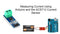The ACS712 is a Hall Effect-based current sensor that can be used to measure AC or DC current. It is a popular choice for use with Arduino because it is easy to use and provides accurate results.

In this blog post, we will show you how to measure current using Arduino and the ACS712 current sensor. We will also discuss the different types of ACS712 current sensors available and how to choose the right one for your needs.

## Types of ACS712 Current Sensors

There are three main types of ACS712 current sensors:

• ACS712EL: The ACS712EL is a low-current sensor that can measure currents up to 5 amps.
• ACS712ELC: The ACS712ELC is a low-current sensor with a center-hole design that makes it easy to install.
• ACS712-20A: The ACS712-20A is a high-current sensor that can measure currents up to 20 amps.

## Choosing the Right ACS712 Current Sensor

When choosing an ACS712 current sensor, it is important to consider the following factors:

• Current range: What is the maximum current that you need to measure?
• Accuracy: How accurate does the measurement need to be?
• Bandwidth: What is the highest frequency of the current that you need to measure?
• Cost: How much are you willing to spend on a current sensor?

### Measuring Current Using Arduino and the ACS712 Current Sensor

To measure current using Arduino and the ACS712 current sensor, you will need the following components:

• Arduino board
• ACS712 current sensor module
• Jumper wires
• Power supply
• Load to measure current (e.g., motor, LED, etc.)

Instructions:

1. Connect the ACS712 current sensor module to the Arduino board.

• Connect the VCC pin on the ACS712 current sensor module to the 5V pin on the Arduino board.
• Connect the GND pin on the ACS712 current sensor module to the GND pin on the Arduino board.
• Connect the OUT pin on the ACS712 current sensor module to an analog input pin on the Arduino board.
2. Connect the load to the ACS712 current sensor module.

Connect the load in series with the ACS712 current sensor module. For example, if you are measuring the current of a motor, you would connect the motor to the ACS712 current sensor module and then connect the other end of the motor to the power supply.

3. Connect the power supply to the Arduino board.

Connect the positive terminal of the power supply to the 5V pin on the Arduino board and the negative terminal to the GND pin.

4. Upload the following code to the Arduino board:

## Example code

The following code shows how to read the voltage from an ACS712 current sensor and convert it to current:

C++
``````#include <Arduino.h>

// Define the analog pin that the ACS712 current sensor module is connected to
const int CURRENT_SENSOR_PIN = A0;

// Define the maximum current that the ACS712 current sensor module can measure
const float MAX_CURRENT = 20.0; // Amps

void setup() {
// Set the analog pin to input mode
pinMode(CURRENT_SENSOR_PIN, INPUT);
}

void loop() {
// Read the voltage from the ACS712 current sensor module

// Convert the voltage to current
float current = (voltage / 1024.0) * MAX_CURRENT;

// Print the current to the serial monitor
Serial.print("Current: ");
Serial.println(current);
delay(1000);
}
``````

Applications of Current Measurement

Current measurement can be used in a variety of applications, such as:

• Monitoring the power consumption of a circuit
• Troubleshooting problems with a circuit
• Optimizing the performance of a circuit
• Designing new circuits
• Measuring the efficiency of power supplies
• Measuring the current consumption of devices

Conclusion

Measuring current using Arduino and the ACS712 current sensor is a simple and straightforward process. With a little bit of effort, you can use Arduino to measure current accurately and reliably.

Here are some additional tips for measuring current using Arduino and the ACS712 current sensor:

• Be sure to use a power supply that can provide enough current for both the Arduino and the ACS712 current sensor.
• If you are measuring high-currents, be sure to use a heatsink on the ACS712 current sensor.
• You can use a calibration factor to improve the accuracy of the current measurement. The calibration factor can be found in the ACS712 current sensor datasheet.
• You can use a library to simplify the process of reading the voltage from the ACS712 current sensor and converting it to current.